# The port

Four ships Berthed in the port. Together sail from a port. The first ship will return to port every two weeks, second ship after 4 weeks, third after 8 weeks and fourth ship after 12 weeks. After how many weeks all the boats gather at the port? How many times have each of the ships appeared in the port in the meantime?

x =  24
a =  12
b =  6
c =  3
d =  2

### Step-by-step explanation:

$a=x\mathrm{/}{t}_{1}=24\mathrm{/}2=12$
$b=x\mathrm{/}{t}_{2}=24\mathrm{/}4=6$
$c=x\mathrm{/}{t}_{3}=24\mathrm{/}8=3$
$d=x\mathrm{/}{t}_{4}=24\mathrm{/}12=2$Did you find an error or inaccuracy? Feel free to write us. Thank you!Tips to related online calculators
Do you want to calculate least common multiple two or more numbers?
Do you want to perform natural numbers division - find the quotient and remainder?
Do you want to convert time units like minutes to seconds?

## Related math problems and questions:

• Three ships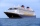There are three ships moored in the port, which sail together. The first ship returns after two weeks, the second after four weeks, and the third after eight weeks. In how many weeks the ships will meet in the port for the first time? How many times have
• Ships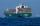Red ship begins its circuit every 30 minutes. Blue boat begins its circuit every 45 minutes. Both ships begin their sightseeing circuit in the same place at the same time always at 10:00 o'clock. a / What time does meet boat again? b / How many times a da
• Three Titanics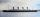Three steamers sailed from the same port on the same day. The first came back on the third day, fourth 4th day and the third returned sixth day. How many days after leaving the steamers met again in the harbor?
• LCMWhat is the least common multiple of 5, 50, 14?
• Two portsBetween the ports of Mumraj and Zmatek, two ships commute along the same route. They spend negligible time in ports, turn around immediately, and continue sailing. At the same time, a blue ship departs from the port of Mumraj, and a green ship departs fro
• PlumsIn the bowl are plums. How many would be there if we can divide it equally among 8, 10 and 11 children?
• Lcm of three numbersWhat is the Lcm of 120 15 and 5
• LCMCommon multiple of three numbers is 3276. One number is in this number 63 times, second 7 times, third 9 times. What are the numbers?
• Tram stop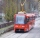The blue tram stops every 12 minutes, the red one 8 minutes. At 8 o'clock they left the stop together. How many times do they meet at a stop before 11 am?
• Bus linesAt 5.00 in the morning, four buses left the terminal at the same time. The first bus has an interval of 15 minutes, the second 20 minutes, the third 25 minutes, and the fourth 45 minutes. How many minutes will all four buses leave the terminal at the same
• Lcm simpleFind least common multiple of this two numbers: 140 175.
• Tram stop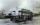At the tram stop met tram No. 4 and no. 5 at 10 AM. Tram no. 4 runs every 5 minutes, tram No. 5 at an interval of 7 minutes. How many times will meet until 12 o'clock?
• Lcm 2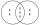Create the smallest possible number that is divisible by numbers 5,8,9,4,3
• Gcd and lcm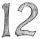Calculate the greatest common divisor and the least common multiple of numbers. a) 16 and 18 b) 24 and 22 c) 45 and 60 d) 36 and 30
• Ships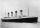At 8:40 the ship set sail at 12 km/h. At 19:10 followed by at 29 km/h sail boat. When sail boat catches up the ship? How many minutes will catch up took?
• Gearing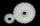Gearing consists of two wheels, one is 88 and second 56 teeth. How many times turns smaller wheel to fit the same teeth as in the beginning? How many times will turn biggest wheel?
• LCM of two numberFind the smallest multiple of 63 and 147